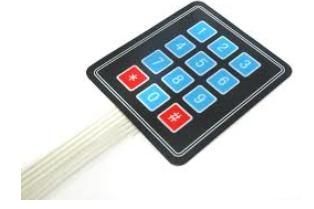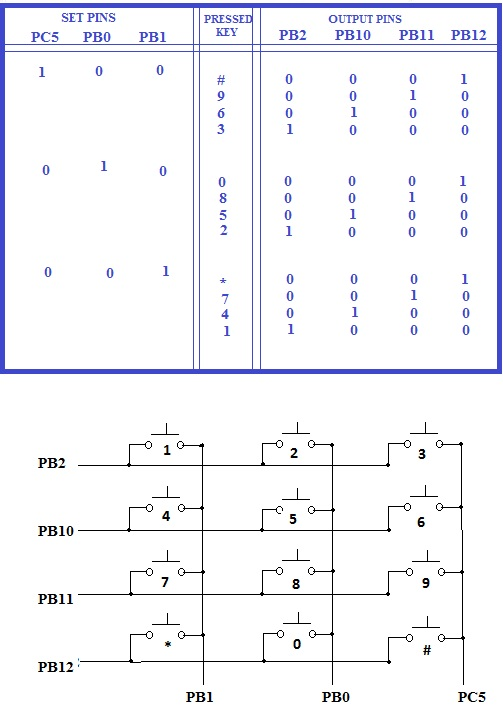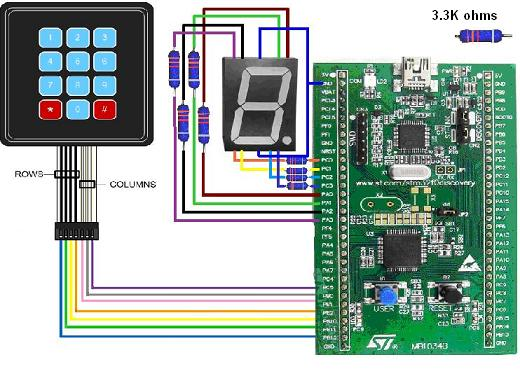Date: 20/09/2014

This module explains how to use 4x3 Membrane Keypad with STM32F0-DISCOVERY microcontroller board

Requirements:

2. Seven segment display & 3K3ohms resistors
3. Connecting wires.
4. General purpose printed circuit board.

Keypad is an array of switches. Matrix keypads are very common input devices in embedded systems. They have simple architecture and are easy to interface. One good thing about them is that they allow you to interface a large number of input keys to a microcontroller with minimum usage of I/O resources. This tutorial type module explains how to read input data from a 3×4 (12 keys) matrix keypad interfaced to an ARM Cortex M0 based STM32F0 microcontroller embedded board and display on a seven segment display. In short this project is to display the pressed key on the seven segment display.Working: There will be 2 wires connected to each switch of the keypad, they get short circuited whenever a button is pressed. For example; when button '1' is pressed, pin PB1 and pin PB2 are connected (as shown in the picture below). Normally when no switch is pressed there is no connection between rows and columns. Whenever a button is pressed contact is made between particular row and column. 4x3 Keypad pin can be directly connected to microcontroller i/o port of this board.Figures above : Keypad logic table and keypad's row and column pins

Keypad Interfacing circuit: The below circuit connects 4x3 Membrane Keypad with port C and port B of microcontroller. The 7-segment display is connected to port C and port B of microcontroller.Programming: The sample C-program/code below displays coresponding numbers pressed on the keypad . The text below in red color-font is the sample code which you can directly use in your compiler.

#include "main.h"
#include "stm32f0xx_conf.h"
uint32_t TickValue=0;

void TimingDelay_Decrement(void)
{
TickValue--;
}

//------------------------------------------------------------------------------
// Function Name : delay_ms
// Description : delay for some time in ms unit(accurate)
// Input : n_ms is how many ms of time to delay
//------------------------------------------------------------------------------
void delay_ms(uint32_t n_ms)
{
// SysTick interrupt each 1000 Hz with HCLK equal to 32MHz
// - 30 to compensate the overhead of this sub routine
SysTick_Config(8000*PLL_MUL_X - 30);
// Enable the SysTick Counter
TickValue = n_ms;
while(TickValue == n_ms)
;
// SysTick interrupt each 1000 Hz with HCLK equal to 32MHz
SysTick_Config(8000*PLL_MUL_X);
while(TickValue != 0)
;
}
void initgpio()
{
GPIO_InitTypeDef GPIO_InitStructure;
RCC_AHBPeriphClockCmd(RCC_AHBPeriph_GPIOA | RCC_AHBPeriph_GPIOB | RCC_AHBPeriph_GPIOC, ENABLE);

GPIO_InitStructure.GPIO_Pin = GPIO_Pin_0 | GPIO_Pin_1 | GPIO_Pin_2 | GPIO_Pin_3 | GPIO_Pin_5 ;
GPIO_InitStructure.GPIO_Speed = GPIO_Speed_10MHz;
GPIO_InitStructure.GPIO_Mode = GPIO_Mode_OUT;
GPIO_InitStructure.GPIO_Otype = GPIO_Otype_PP;
GPIO_Init(GPIOC, &GPIO_InitStructure);

GPIO_InitStructure.GPIO_Pin = GPIO_Pin_0 | GPIO_Pin_1 ;
GPIO_InitStructure.GPIO_Speed = GPIO_Speed_10MHz;
GPIO_InitStructure.GPIO_Mode = GPIO_Mode_OUT;
GPIO_InitStructure.GPIO_Otype = GPIO_Otype_PP;
GPIO_Init(GPIOB, &GPIO_InitStructure);

GPIO_InitStructure.GPIO_Pin = GPIO_Pin_0 | GPIO_Pin_1 | GPIO_Pin_2 | GPIO_Pin_3 ;
GPIO_InitStructure.GPIO_Speed = GPIO_Speed_10MHz;
GPIO_InitStructure.GPIO_Mode = GPIO_Mode_OUT;
GPIO_InitStructure.GPIO_Otype = GPIO_Otype_PP;
GPIO_Init(GPIOA, &GPIO_InitStructure);

GPIO_InitStructure.GPIO_Pin = GPIO_Pin_2 | GPIO_Pin_10 | GPIO_Pin_11 | GPIO_Pin_12;
GPIO_InitStructure.GPIO_Speed = GPIO_Speed_10MHz;
GPIO_InitStructure.GPIO_Mode = GPIO_Mode_IN;
GPIO_InitStructure.GPIO_Otype = GPIO_Otype_PP;
GPIO_InitStructure.GPIO_PuPd = GPIO_PuPd_DOWN;
GPIO_Init(GPIOB, &GPIO_InitStructure);
}
void display(int b)
{
switch(b)
{
case 0: GPIOA->ODR = 0x0007; //0
GPIOC->ODR = 0x0007;
delay_ms(1000);
break;
case 1:
GPIOA->ODR = 0x0001; //1
GPIOC->ODR = 0x0004;
delay_ms(1000);
break;
case 2:
GPIOA->ODR = 0x000B; //2
GPIOC->ODR = 0x0003;
delay_ms(1000);
break;
case 3:
GPIOA->ODR = 0x000B; //3
GPIOC->ODR = 0x0006;
delay_ms(1000);
break;
case 4:
GPIOA->ODR = 0x000D; //4
GPIOC->ODR = 0x0004;
delay_ms(1000);
break;
case 5:
GPIOA->ODR = 0x000E; //5
GPIOC->ODR = 0x0006;
delay_ms(1000);
break;
case 6:
GPIOA->ODR = 0x000E; //6
GPIOC->ODR = 0x0007;
delay_ms(1000);
break;
case 7:
GPIOA->ODR = 0x0003; //7
GPIOC->ODR = 0x0004;
delay_ms(1000);
break;
case 8:
GPIOA->ODR = 0x000F; //8
GPIOC->ODR = 0x0007;
delay_ms(1000);
break;
case 9:
GPIOA->ODR = 0x000F; //9
GPIOC->ODR = 0x0006;
delay_ms(1000);
break;
case 10:
GPIOA->ODR = 0x000F; //*
GPIOC->ODR = 0x0000;
delay_ms(1000);
break;
case 11:
GPIOA->ODR = 0x0008; //#
GPIOC->ODR = 0x000F;
delay_ms(1000);
break;
}
return ;
}

int main(void)
{

initgpio();

while(1)
{
GPIOC->BSRR = GPIO_Pin_5;//set bit as high
GPIOB->BRR = GPIO_Pin_0;//set bit as low
GPIOB->BRR = GPIO_Pin_1;//set bit as low
{
display(3);
display(6);
display(9);
display(11);
}
GPIOC->BRR = GPIO_Pin_5;//set bit as low
GPIOB->BSRR = GPIO_Pin_0;//set bit as high
GPIOB->BRR = GPIO_Pin_1;//set bit as low
{
display(2);
display(5);
display(8);
display(0);

}
GPIOC->BRR = GPIO_Pin_5;//set bit as low
GPIOB->BRR = GPIO_Pin_0;//set bit as low
GPIOB->BSRR = GPIO_Pin_1;//set bit ashigh
{
display(1);
display(4);
display(7);
display(10);

}

}

}

Click below to visit next and other embedded system course modules

Module 9: Controller Area Networking
(CAN)

Module 12: SPI Bus Interface

Module14: USB Interface

Module15: SRAM memory interface

Module16: Flash memory interface

Module17: LCD display panel interface

Module18: Touch pane interface

Module19: Audio/video interface

Previous Modules

Module 5b: ARM Cotex M0
Architecture

Author: Srinivasa Reddy N